Next: Exercises Up: Multipole Expansion Previous: Spherical Wave Expansion of

# Mie Scattering

Consider a plane electromagnetic wave incident on a spherical obstacle. In general, the wave is scattered, to some extent, by the obstacle. Thus, far away from the sphere, the electromagnetic fields can be expressed as the sum of a plane wave and a set of outgoing spherical waves. There may be absorption by the obstacle, as well as scattering. In this case, the energy flow away from the obstacle is less than the total energy flow towards it--the difference representing the absorbed energy.

The fields outside the sphere can be written as the sum of incident and scattered waves: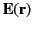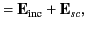(1588)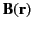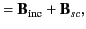(1589)

where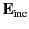and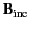are given by (1588)-(1589). Because the scattered fields are outgoing waves at infinity, their expansions must be of the form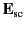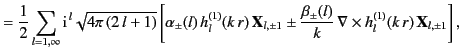(1590)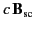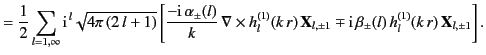(1591)

The coefficients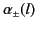and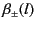are determined by the boundary conditions on the surface of the sphere. In general, it is necessary to sum over all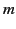harmonics in the above expressions. However, for the restricted class of spherically symmetric scatterers, only the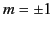harmonics need be retained (because only these harmonics occur in the spherical wave expansion of the incident plane wave [see Equations (1588)-(1589)], and a spherically symmetric scatterer does not couple differentharmonics).

The angular distribution of the scattered power can be written in terms of the coefficients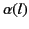and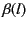using the scattered electromagnetic fields evaluated on the surface of a sphere of radius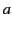surrounding the scatterer. In fact, it is easily demonstrated that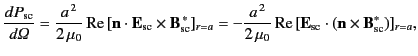(1592)

where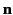is a radially directed outward normal. The differential scattering cross-section is defined as the ratio of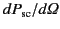to the incident flux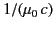. Hence,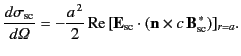(1593)

We need to evaluate this expression using the electromagnetic fields specified in Equations (1588)-(1593). The following identity, which can be established with the aid of Equations (1438), (1476), and (1486), is helpful in this regard: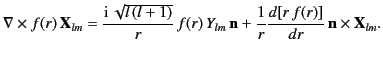(1594)

For instance, using this result, we can write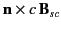in the form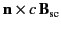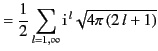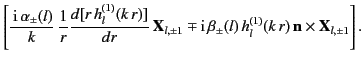(1595)

It can be demonstrated, after considerable algebra, that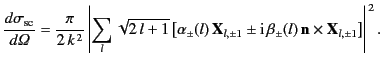(1596)

In obtaining this formula, use has been made of the standard result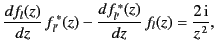(1597)

where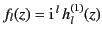. The total scattering cross-section is obtained by integrating Equation (1598) over all solid angle, making use of the following orthogonality relations for the vector spherical harmonics [see Equations (1477)-(1478)]: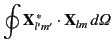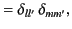(1598)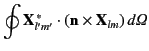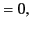(1599)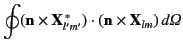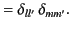(1600)

Thus,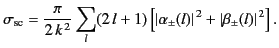(1601)

According to Equations (1598) and (1603), the total scattering cross-section is independent of the polarization of the incident radiation (i.e., it is the same for both the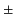signs). However, the differential scattering cross-section in any particular direction is, in general, different for different circular polarizations of the incident radiation. This implies that if the incident radiation is linearly polarized then the scattered radiation is elliptically polarized. Furthermore, if the incident radiation is unpolarized then the scattered radiation exhibits partial polarization, with the degree of polarization depending on the angle of observation.

The total power absorbed by the sphere is given by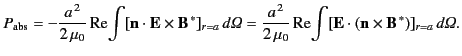A similar calculation to that outlined above yields the following expression for the absorption cross-section,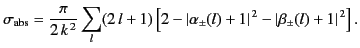(1602)

The total, or extinction, cross-section is the sum of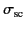and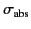: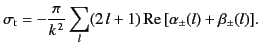(1603)

Not surprisingly, the above expressions for the various cross-sections closely resemble those obtained in quantum mechanics from partial wave expansions.

Let us now consider the boundary conditions at the surface of the sphere (whose radius is, say). For the sake of simplicity, let us suppose that the sphere is a perfect conductor. In this case, the appropriate boundary condition is that the tangential electric field is zero at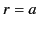. According to Equations (1588), (1590), and (1596), the tangential electric field is given by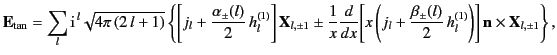(1604)

where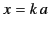, and all of the spherical Bessel functions have the argument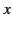. Thus, the boundary condition yields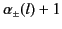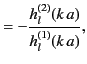(1605)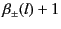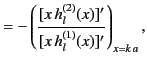(1606)

where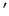denotes. Note that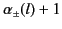andare both numbers of modulus unity. This implies, from Equation (1604), that there is no absorption for the case of a perfectly conducting sphere (in general, there is some absorption if the sphere has a finite conductivity). We can writeandin the form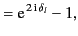(1607)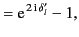(1608)

where the phase angles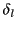and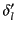are called scattering phase shifts. It follows from Equations (1607)-(1608) that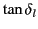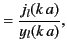(1609)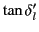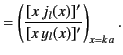(1610)

Let us specialize to the limit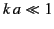, in which the wavelength of the radiation is much greater than the radius of the sphere. The asymptotic expansions (1428)-(1429) yield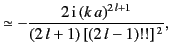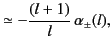(1611)

for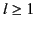. It is clear that the scattering coefficientsandbecome small very rapidly as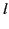increases. Thus, in the very long wavelength limit, only the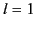coefficients need be retained. It is easily seen that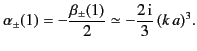(1612)

In this limit, the differential scattering cross-section (1598) reduces to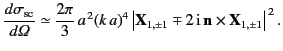(1613)

It can be demonstrated that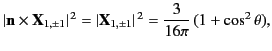(1614)

and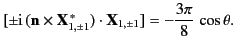(1615)

Thus, in long wavelength limit, the differential scattering cross-section reduces to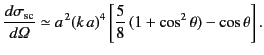(1616)

The scattering is predominately backwards, and is independent of the state of polarization of the incident radiation. The total scattering cross-section is given by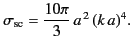(1617)

This well-known result was first obtained by Mie and Debye. Note that the cross-section scales as the inverse fourth power of the wavelength of the incident radiation. This scaling is generic to all scatterers whose dimensions are much smaller than the wavelength. In fact, it was first derived by Rayleigh using dimensional analysis.Next: Exercises Up: Multipole Expansion Previous: Spherical Wave Expansion of
Richard Fitzpatrick 2014-06-27